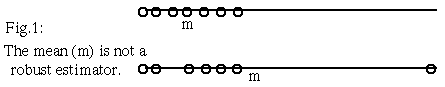Robust Estimators of Location

a project for Pattern Recognition cs644 taught by prof. Toussaint at McGill University.

by

Greg Aloupis

IMPORTANT NOTEFig.0 : image of nice location (unrelated to topic).

Introduction

(What is an estimator of location?)

Consider the following problem: you are given a set of data which represents measurements of some quantity. Your task is to estimate the true value (location) of the measured quantity. In other words, given a set of points in Rd, find a point which best describes the set. If you find this problem interesting, or better yet, if you believe that this problem is trivial, then you should definitely keep reading.

(What do we mean by "robust"?)

When dealing with problems such as the above, it is important to consider the issue of robustness: how much does the answer change if some of the data is perturbed? How easy is it to create a data set for which an estimator yields an absurd answer? As an example of a non-robust estimator (which also shows why the problem is not trivial), take the mean (or center of mass) of the set of points: if one point is moved "far" from the rest, the mean will follow (fig.1). To be literally extreme, if we move the "corrupt" point to infinity, the mean will also go to infinity. This example indicates that robustness is particularly important when there is a possibility that our data set contains "contaminated" or "corrupt" data. It is also important when we simply do not want some data to have more importance than others. If we take the mean as an estimator, outlying points carry more "weight" than points near the mean. In fig.1, deleting the outlying point would have a greater impact on the location of the mean than deleting a point in the dense region.A note: In this discussion, we are dealing with non-parametric distributions of data points.

Robustness in More Detail

As mentioned above, a single point is enough to greatly influence the mean of a data set. By contrast, at least 50% of a data set must be moved to infinity in order to force the median to do the same. This suggests a measure of robustness for estimators of location:

The BREAKDOWN POINT is the proportion of data which must be moved to infinity so that the estimator will do the same.
Thus in 1D (univariate data set) the median has a breakdown point of 1/2 and the mean has a breakdown point of 1/n, where n is the number of data. It has been shown that the maximum breakdown point for an estimator is 1/2, so in 1D, the median excels according to this robustness criterion.

Other measures of robustness, typically used in 2D or higher, include invariance to certain types of transformations of the data. For example, take a set of points in the plane, R2. We would not want our estimator to be affected by the choice of coordinate axes (including origin, direction and scale).

The Median as an Estimator of Location

We have already seen that the high breakdown point of the median in 1D is a reason for choosing it over the mean as an estimator of location. Clearly, the breakdown point of the mean is 1/n in all dimensions, as you may visualize in 2D or 3D. If we attempt to make a similar statement about the median, we immediately face a problem: what is the median in dimensions higher than one? This question has been asked by statisticians for at least a century, and the answer is still not clear. There is more than one way to describe the univariate median (keep reading to find out), and therefore there are many multivariate theories which reduce to the well known results of 1D.

Naive multivariate medians

Clearly, we cannot generalize the 1D median to higher dimensions by arbitrarily selecting a direction in the high-dimensional space. Some people suggest combining various medians taken in different directions. In 1902, Hayford suggested the vector-of-medians of orthogonal coordinates. This is similar to finding the multivariate mean, and works well for other problems such as finding derivatives of functions (gradient). Unfortunately, as Hayford was aware, this solution is dependent on the choice of orthogonal directions. You may verify this easily using a set of three non-collinear points. As a "master" of naive approaches, I considered taking the median in the O(n2) directions between all points of the set. This seems to be robust to rigid transformations. However it does not generalize from the univariate median (in other words, it's just a "hack"). Please let me know if you have seen this idea before, or if you have any interesting examples of data that make it fail.

The following sections describe certain definitions of multivariate medians that have been proposed, in no particular order (see Small'90 for more detail).

next : the L1 median